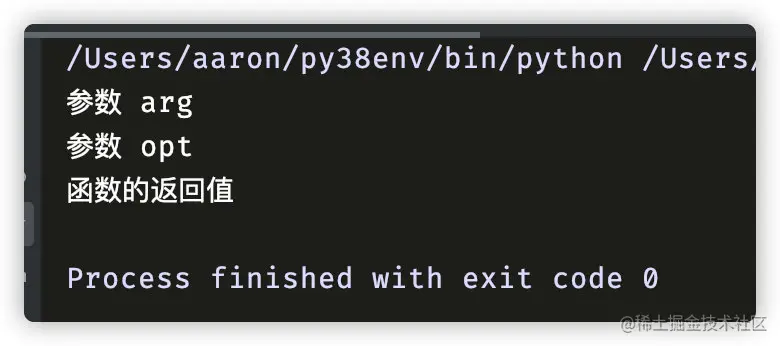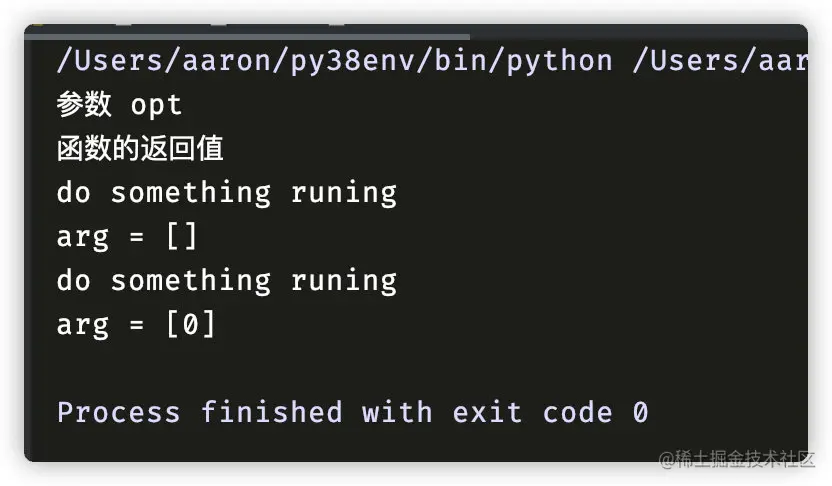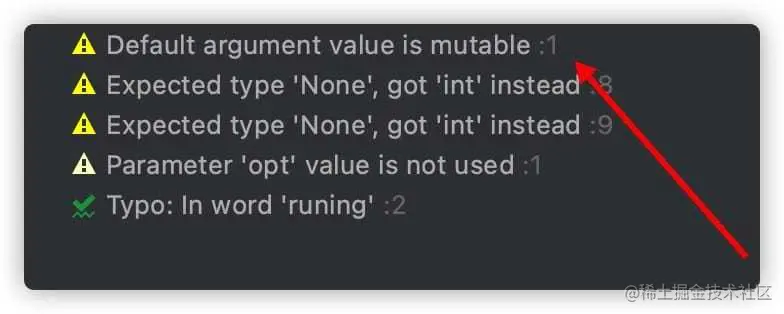# The unknown side of Python functions

2022-01-31 09:52:14 somenzz

Usually we define a function , Then the function is called. , The code related to the function begins to execute . But many people don't know , When we define a function , Some code starts to execute . Today, let's talk about the unknown side of functions .

Let's start with a piece of code ：

``````def do_something(opt: print(' Parameters  opt'), arg=print(' Parameters  arg')) -> print(' The return value of the function '):
print("do something runing")

if __name__ == '__main__':
pass
Copy code ``````

In the above code, we set a function , But it was not called , Does it output information ？

It will be , In the parameter, whether it is the type prompt part , Or the default assignment part , Or the type prompt of the return value , The statements inside will be executed , give the result as follows ：This practice is very rare for me , In fact print Open file instead of function , Connect to the database and other operations , Will also be executed .

But usually , Parameters are immutable types , If you pass in a variable type , Maybe every function call , The results will be changed , for example ：

``````
def do_something(opt: print(" Parameters  opt"), arg=[]) -> print(" The return value of the function "):
print("do something runing")
print(f"{arg = }")
arg.append(0)

if __name__ == "__main__":
do_something(opt=1)
do_something(opt=1)

Copy code ``````

The operation results are as follows ：You can find , Call the function twice do_something, Although not introduced arg Parameters ,arg The value of has sent a change . If you don't pay attention to this , There may be a bug happen . stay Pythcarm in , Will warn us arg It's a mutable object ：If you want to get the type prompt of the function 、 The default value is , It can be like this ：

``````def do_something(opt: 1, arg=2) -> 3:
print("do something runing")
print(f"{arg = }")
arg.append(0)

if __name__ == "__main__":
print(f"{do_something.__annotations__ = }")
print(f"{do_something.__defaults__ = }")

#do_something.__annotations__ = {'opt': 1, 'return': 3}
#do_something.__defaults__ = (2,)
Copy code ``````

## Last

This article shares Python The unknown side of functions , If there is a harvest , Please thumb up 、 Looking at 、 Focus on supporting .